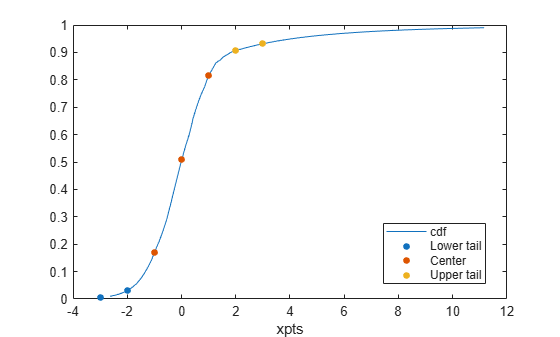# segment

Piecewise distribution segments containing input values

## Syntax

``s = segment(pd,x)``
``s = segment(pd,[],p)``

## Description

example

````s = segment(pd,x)` returns a vector `s` of positive integers indicating which segment in the piecewise distribution `pd` contains each quantile value in `x`.The values 1, 2, and 3 in `s` indicate the lower tail, center, and upper tail segments in `pd`, respectively. If `pd` does not include a lower tail segment, then 1 and 2 indicate the center and upper tail segments, respectively.```

example

````s = segment(pd,[],p)` returns a vector `s` of positive integers indicating which segment in the piecewise distribution `pd` contains each cumulative probability value in `p`.```

## Examples

collapse all

Generate a sample data set and create a `paretotails` object by fitting a piecewise distribution with Pareto tails to the generated data. Find the segment containing the specified quantile values by using the object function `segment`.

Generate a sample data set containing 20% outliers.

```rng('default'); % For reproducibility left_tail = -exprnd(1,100,1); right_tail = exprnd(5,100,1); center = randn(800,1); x = [left_tail;center;right_tail];```

Create a `paretotails` object by fitting a piecewise distribution to x. Specify the boundaries of the tails using the lower and upper tail cumulative probabilities so that a fitted object consists of the empirical distribution for the middle 80% of the data set and generalized Pareto distributions (GPDs) for the lower and upper 10% of the data set.

`pd = paretotails(x,0.1,0.9)`
```pd = Piecewise distribution with 3 segments -Inf < x < -1.33251 (0 < p < 0.1): lower tail, GPD(-0.0063504,0.567017) -1.33251 < x < 1.80149 (0.1 < p < 0.9): interpolated empirical cdf 1.80149 < x < Inf (0.9 < p < 1): upper tail, GPD(0.24874,3.00974) ```

Find the segment containing the specified points by using the `segment` function.

```xpts = -3:3; s = segment(pd,xpts)```
```s = 1×7 1 1 2 2 2 3 3 ```

1, 2, and 3 indicate the lower tail, center, and upper tail segments in `pd`, respectively.

Draw the scatter plot of the points (`xpts`) grouped by their segments over the cumulative distribution function (cdf) plot. Plot the cdf of `pd`.

```xgrid = linspace(icdf(pd,.01), icdf(pd,.99)); ygrid = cdf(pd,xgrid); plot(xgrid,ygrid)```

Superimpose the scatter plot of `xpts` by using `gscatter`.

```hold on gscatter(xpts,cdf(pd,xpts),s) legend('cdf','Lower tail','Center','Upper tail') hold off```Generate a sample data set and create a `paretotails` object by fitting a piecewise distribution with Pareto tails to the generated data. Find the segment containing the boundary points by using the object function `segment`.

Generate a sample data set containing 20% outliers.

```rng('default'); % For reproducibility left_tail = -exprnd(1,100,1); right_tail = exprnd(5,100,1); center = randn(800,1); x = [left_tail;center;right_tail];```

Create a `paretotails` object by fitting a piecewise distribution to `x`. Specify the boundaries of the tails using the lower and upper tail cumulative probabilities so that a fitted object consists of the empirical distribution for the middle 80% of the data set and generalized Pareto distributions (GPDs) for the lower and upper 10% of the data set.

`pd = paretotails(x,0.1,0.9)`
```pd = Piecewise distribution with 3 segments -Inf < x < -1.33251 (0 < p < 0.1): lower tail, GPD(-0.0063504,0.567017) -1.33251 < x < 1.80149 (0.1 < p < 0.9): interpolated empirical cdf 1.80149 < x < Inf (0.9 < p < 1): upper tail, GPD(0.24874,3.00974) ```

Return the boundary values between the piecewise segments by using the `boundary` function.

`[p,q] = boundary(pd)`
```p = 2×1 0.1000 0.9000 ```
```q = 2×1 -1.3325 1.8015 ```

The values in `p` are the cumulative probabilities at the boundaries, and the values in `q` are the corresponding quantiles.

Find the segment containing the boundary points by using the quantile values.

`s1 = segment(pd,q)`
```s1 = 2×1 2 3 ```

1, 2, and 3 indicate the lower tail, center, and upper tail segments in `pd`, respectively. The output `s1` implies that the first boundary between the lower tail segment and the center segment belongs to the center segment, and the second boundary between the center segment and the upper tail segment belongs to the upper tail segment.

You can also use the cumulative probability values to find the corresponding segments.

`s2 = segment(pd,[],[0;p;1])`
```s2 = 4×1 1 2 3 3 ```

## Input Arguments

collapse all

Piecewise distribution with Pareto tails, specified as a `paretotails` object.

Quantile values, specified as a numeric vector.

Data Types: `single` | `double`

Cumulative probability values, specified as a numeric vector of range `[0,1]` values.

Data Types: `single` | `double`

Introduced in R2007a